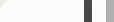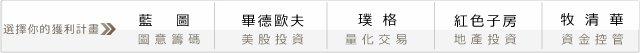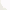## 你拿生命在交易，還是把交易當生命?

#### 模擬0.2R期望值的賭局50,000次，觀察其損益分布!

0.2R期望值的賭局，有很多種Cases。下面皆為0.2R期望值的賭局。

1. R-multiple為1R，勝率為 60% (期望損益：60%*1R + 40%*(-R) = 0.2R)
2. R-multiple為2R，勝率為 40% (期望損益：40%*2R + 60%*(-R) = 0.2R)
3. R-multiple為3R，勝率為 30% (期望損益：30%*3R + 70%*(-R) = 0.2R)
4. R-multiple為4R，勝率為 24% (期望損益：24%*4R + 76%*(-R) = 0.2R)
5. R-multiple為5R，勝率為 20% (期望損益：20%*4R + 80%*(-R) = 0.2R)

#### 高勝率、低賠率策略好嗎?

1. R-multiple為0.8R，勝率為 66% (期望損益：66%*0.8R + 34%*(-R) = 0.2R)
2. R-multiple為0.7R，勝率為 71% (期望損益：71%*0.7R + 29%*(-R) = 0.2R)
3. R-multiple為0.6R，勝率為 75% (期望損益：75%*0.6R + 25%*(-R) = 0.2R)
4. R-multiple為0.5R，勝率為 80% (期望損益：80%*0.5R + 20%*(-R) = 0.2R)

#### 拿生命在交易，隨時有陣亡的可能

(註：本篇文章感謝Paucky大力幫忙，實驗與討論!)

#### 0 意見: